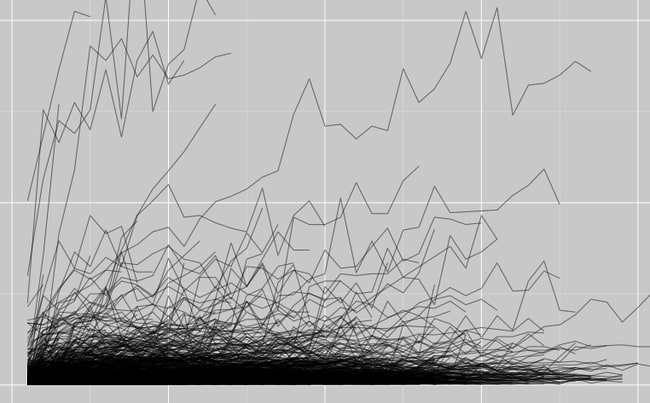# Cohort Analysis for Startups&#58; Six Summary Reports to Understand Your Customer Base (With Code)Cohort analysis provides deep insight into customer bases because cohorts expose how customer accounts grow, evolve and churn. Plus, cohort analysis provides a framework to evaluate product releases, marketing pushes and advertising campaign performance.

## The Six Cohort Reports I Use to Understand Trends

Below is a list of the six reports I create when analyzing cohorts. The combination of these six reports provides a robust summary of the state of a customer base. By no means exhaustive, this collection is a solid starting point and of course these are framed in the context of revenue but could easily be changed to activity or another metric.

1. Average Revenue Per Customer Over Time - Chart monthly revenue over time to contrast with cohort data
2. Individual Account Growth Over Time - Chart all accounts to visualize trends.
3. Typical Account Growth Over Time - Chart how the average account grows with time
4. Number of Customers in Each Cohort - Chart number of customers in each cohort to see how sensitive cohort data is to sample size and also see the size of the new customer pipeline over time.
5. Average Monthly Revenue By Cohort - Chart the revenue by cohort to see if newer customers generate more or less revenue than older customers. Really good for marketing spend evaluation.
6. Cohort Comparison - Chart the different cohorts over time to see how their revenue characteristics compare.

## Implementation in R

Cohort analysis is difficult to perform in a database or in Excel so I turned to R. Below is a generic cohort analysis script for R, the open source statistical language.

I use two libraries by Hadley Wickham, plyr for data manipulation and ggplot2 for plotting the data. Hadley is at the forefront of R and an extraordinary contributor to the community. I can’t overstate his impact on the way I use R. I seem to use only standard libraries and Hadley’s.

To use this data analysis, start with a file with 3 columns: “date” column (YYYY-MM-DD), “company_name” column, monthly revenue “revenue”. If your file isn’t in this format, use Hadley’s reshape library to “melt” the data. Note: I use tab-separated files but you could easily use a CSV.

If you have ideas for improving the script or if you’ve found a bug, send me a note using the email link on the left.

``````   ### IMPORT LIBRARIES ###
library(plyr)
library(ggplot2)
library(lubridate)

### IMPORT DATA ###
account_data <- read.delim("data.txt")
account_data \$date = as.Date(account_data\$date)

### COMPUTE AVERAGES ACROSS CUSTOMER BASE ###
monthly_average = ddply(account_data,~date,summarise, avg_revenue=mean(revenue), customers = length(unique(company_name)))

ggplot(monthly_average, aes(x=date, y=avg_revenue)) + geom_line() + labs(title = "Average revenue by Calendar Month for Customers in \$", x="Year", y="Average revenue (\$)")

### REVENUE BY COHORT ###
cohort = ddply(account_data,~company_name,summarise,cohort=min(date), revenue=sum(revenue), num_months_as_customer = as.numeric(round((as.Date(format(Sys.time(), "%Y-%m-%d")) - min(date))/(365.25/12)) + 1))
cohort_summary = ddply(cohort, ~cohort, summarise, mean_revenue = sum(revenue)/sum(num_months_as_customer) )
ggplot(cohort_summary) + geom_bar(aes(cohort, mean_revenue), stat="identity") + labs(title="Average Monthly Revenue by Cohort", x="Cohort", y="Monthly Revenue")

### COHORT ANALYSIS -- PLOT INDIVIDUAL ACCOUNT GROWTH OVER TIME, USE ALPHA TO EXTRACT PATTERNS ###
accounts= ddply(account_data, .(company_name), transform, cmonth = as.numeric(round((date - min(date))/(365.25/12)) + 1, cohort = min(date)))
ggplot(accounts, aes(x=cmonth, y=revenue, group=company_name)) + geom_line(alpha=0.3) + labs(title="Individual Account Growth Over Time", x = "Months Since Becoming a Paid Customer", y = "Monthly Recurring Revenue")

### COHORT ANALYSIS - PLOT THE AVERAGE ACCOUNT GROWTH OVER TIME ###
average_growth = ddply(accounts, .(cmonth), summarise, avg_revenue = mean(revenue), num = length(company_name))
ggplot(average_growth) + geom_line(aes(cmonth,avg_revenue))  + labs(title="Account Growth Over Time", x = "Months Since Becoming a Paid Account", y = "Monthly Recurring Revenue (\$)")

### PLOT THE NUMBER OF CUSTOMERS IN EACH COHORT ###
ggplot(average_growth) + geom_bar(aes(x=cmonth, y=num), stat="identity") + labs(title = "Number of Customers in Each Cohort", x="Months Since Becoming a Paid Customer", y = "Customers")

### PLOT THE AVERAGE revenue OF CUSTOMERS BY DATE JOINED ###
account_data = ddply(account_data, .(company_name), transform, cohort = min(date))
agg = ddply(account_data, .(cohort), summarise, avg_revenue = mean(revenue))
ggplot(agg, aes(x = cohort, y=avg_revenue)) + geom_bar(stat="identity") + labs(title = "Average revenue of Customers by Date Joined", x = "Date Became a Paid Customer", y = "Average revenue (\$)")

### COMPARE COHORT REVENUE OVER TIME ###
ggplot(account_data) + geom_line(aes(x=date, y=revenue, group=cohort, colour=cohort))
``````

# Tomasz Tunguz

Venture Capitalist at Redpoint

© Tomasz Tunguz

2019